# “To iterate is human, to recurse, divine.” – A guide to RecursionPrerequisites:

• Logical progression of thought
• Some mathematical aptitude
• Basic knowledge of functions

Recursion is possibly one of the most challenging concepts in Computer Science to get the hang of, and something which has fascinated me for a very long time. Sure, we all know the textbook definition:

“Recursion is a method where the solution to a problem depends on the solutions to smaller instances of the same problem.”

But it still takes an “Aha! Moment” to get an intuitive grasp on it, which is why I’ll finally take a stab at it.

As usual, we have three levels, so switch to them as you see fit:

Algosaurus coincidentally happens to be part of an obscure death metal band you’ve never heard of. He goes to a concert venue with a poorly-designed audio system and starts off with his smash-hit song “Rock Tore My World Apart”. He growls into the mic as he descends into the chorus and…

…is rudely interrupted by a loud screeching sound from the amplifier.What just happened was a feedback loop. While Algosaurus was singing (growling?) his heart out, his mic picked up the output of the surrounding amplifiers, and fed it right back into the input.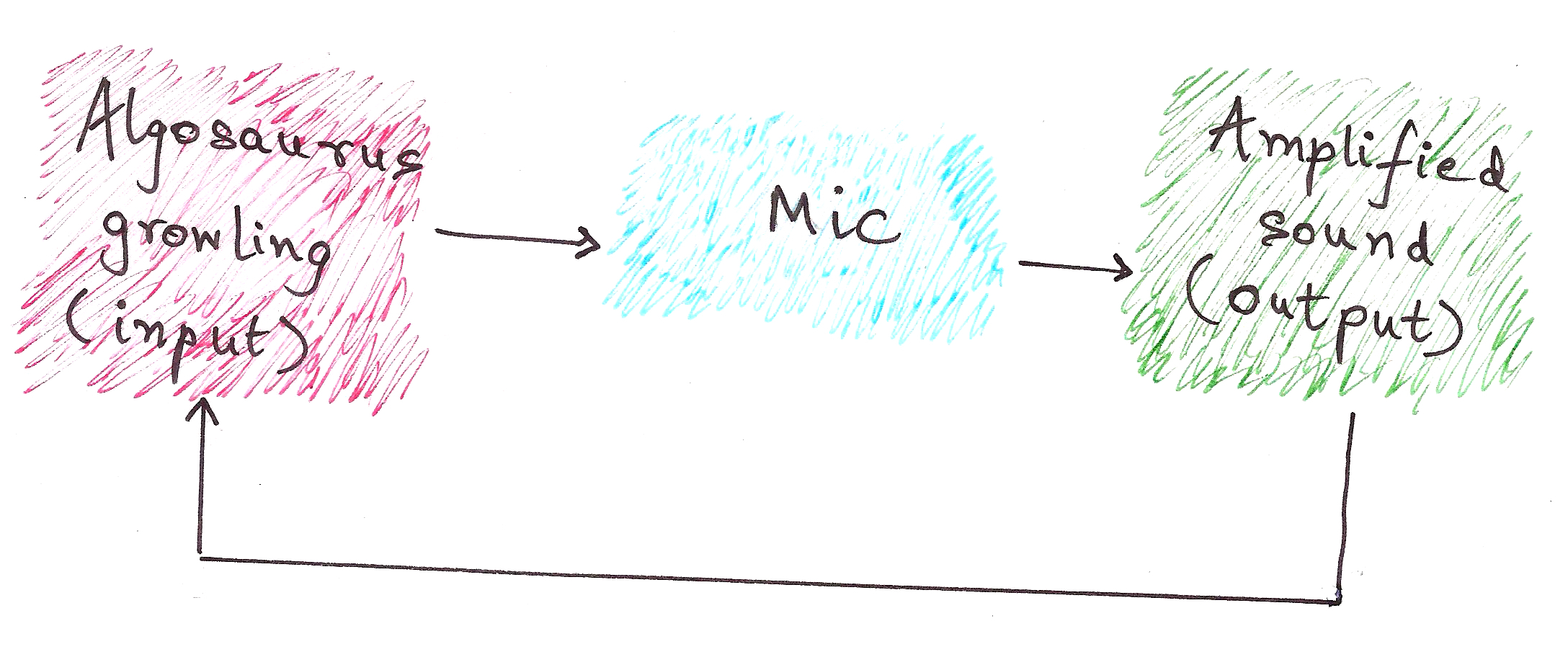Although the above example doesn’t reduce to a meaningful output, rather it results in a stack overflow (the screeching sound), this is the essential idea of recursion.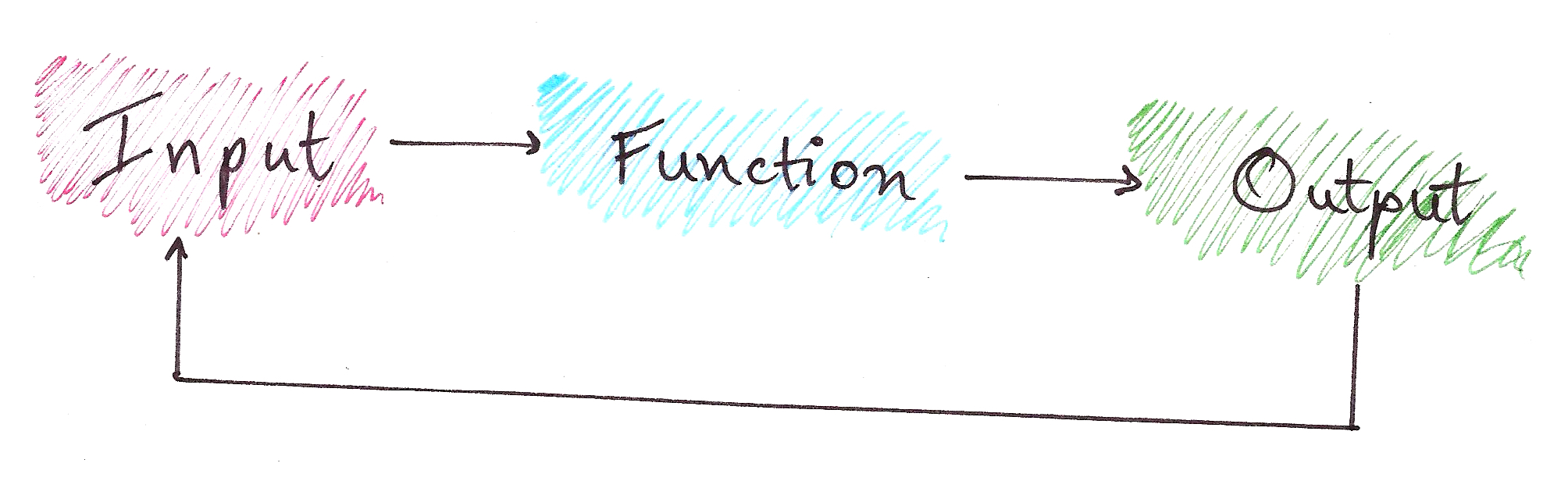In recursion, we have:

Problem
|
Sub-problem (which is Problem with a smaller input)
|
Base Case (a Problem with a constant output)

Confused by the jargon?So was I.

No article on recursion is complete without talking about Fibonacci Numbers.

Fibonacci series: 0, 1, 1, 2, 3, 5, 8, 13, 21…

You’ll notice that the nth Fibonacci number, after n = 0 and n = 1, is the sum of the previous two Fibonacci numbers.

Namely,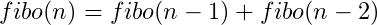Notice how this is a recursive definition? Let’s break it down into a recursion tree.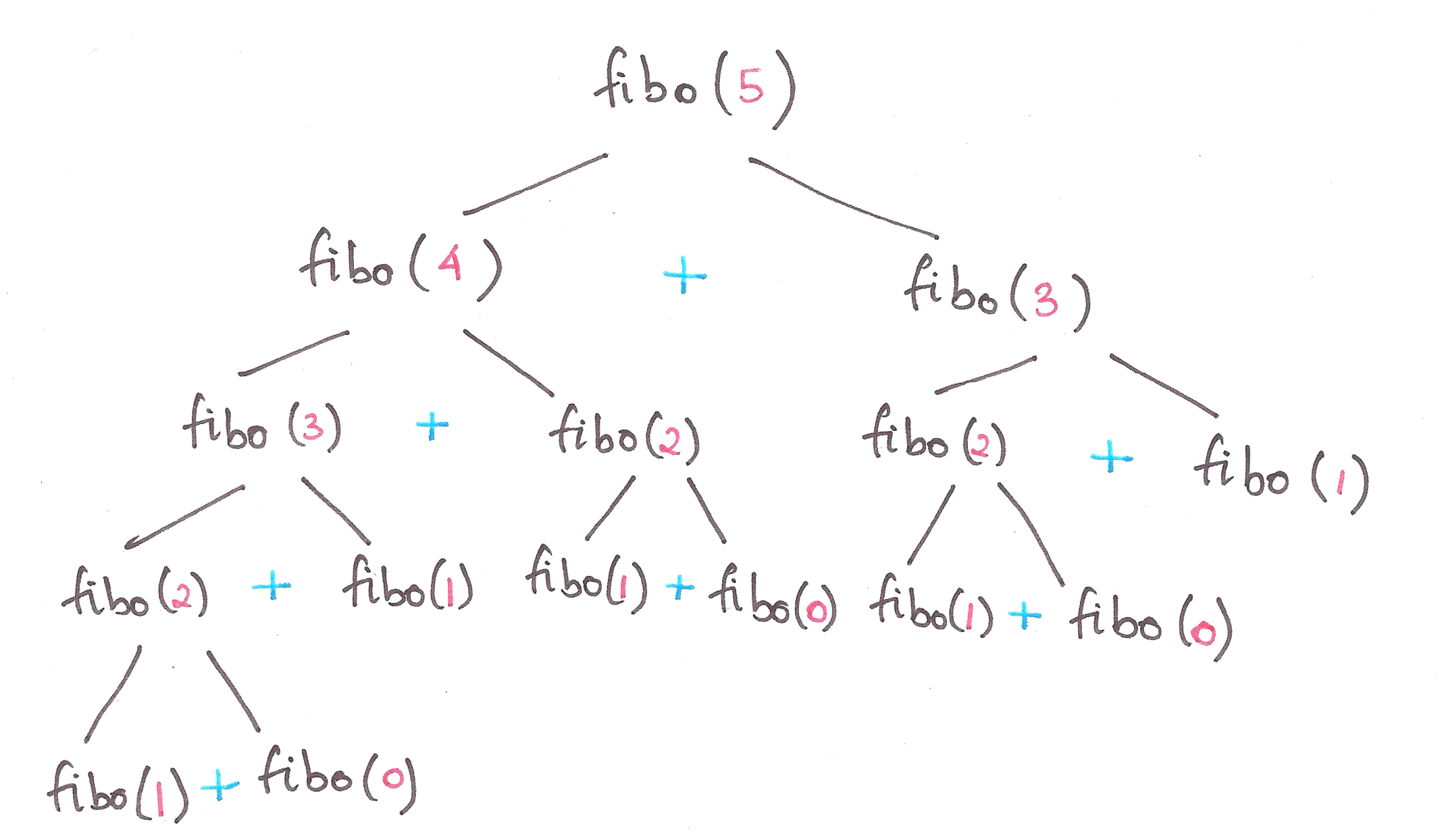So, let’s define Fibonacci numbers in terms of Problems and Base Cases.

Problem:|
Sub-problem(s):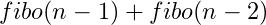|
Constant Base Case: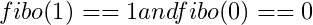This is also known as the divide-and-conquer paradigm of algorithms, where we segregate a problem into smaller sub-problems, and finally into a simple base case.

Final code for Fibonacci numbers?

```def fibo(n):
if n == 0:
result = 0 #Base case
elif n == 1:
result = 1 #Base case
else:
result = fibo(n-1) + fibo(n-2) #Feeding the 'output' right back into the input function
return result
```Lo and behold, you just learnt recursion.

Let’s take another classic example to hit the point home.

Once upon a time, in the Cretaceous Era, there lived a family of Algosauri in a land far, far away. Baby Algosaurus had a toy with 3 rods and 7 discs.His Dad challenged him to a game, where the objective was to transfer all the discs to another rod, obeying the following rules:

1. Only one disk may be moved at a time.
2. Only the uppermost disk from one rod can be moved at once.
3. It can be put on another rod, only if that rod is empty or if the uppermost disk of that rod is larger than the disc being moved.

How did Baby Algosaurus do it?

Let’s assume there to be n number of discs, and we’ll be moving the tower from source to target using the helper rod:

For n = 1: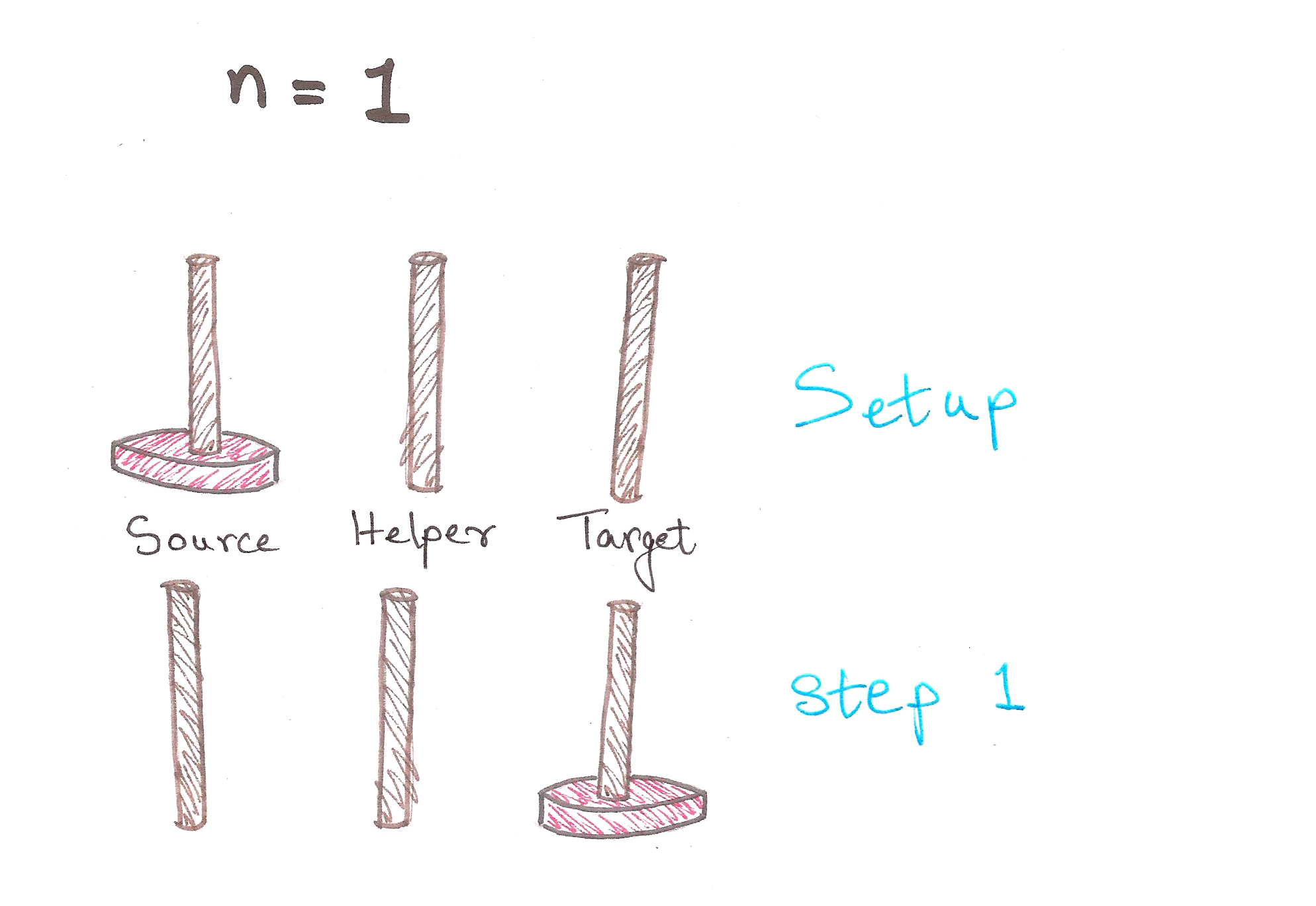1 step. Easy enough.

For n = 2: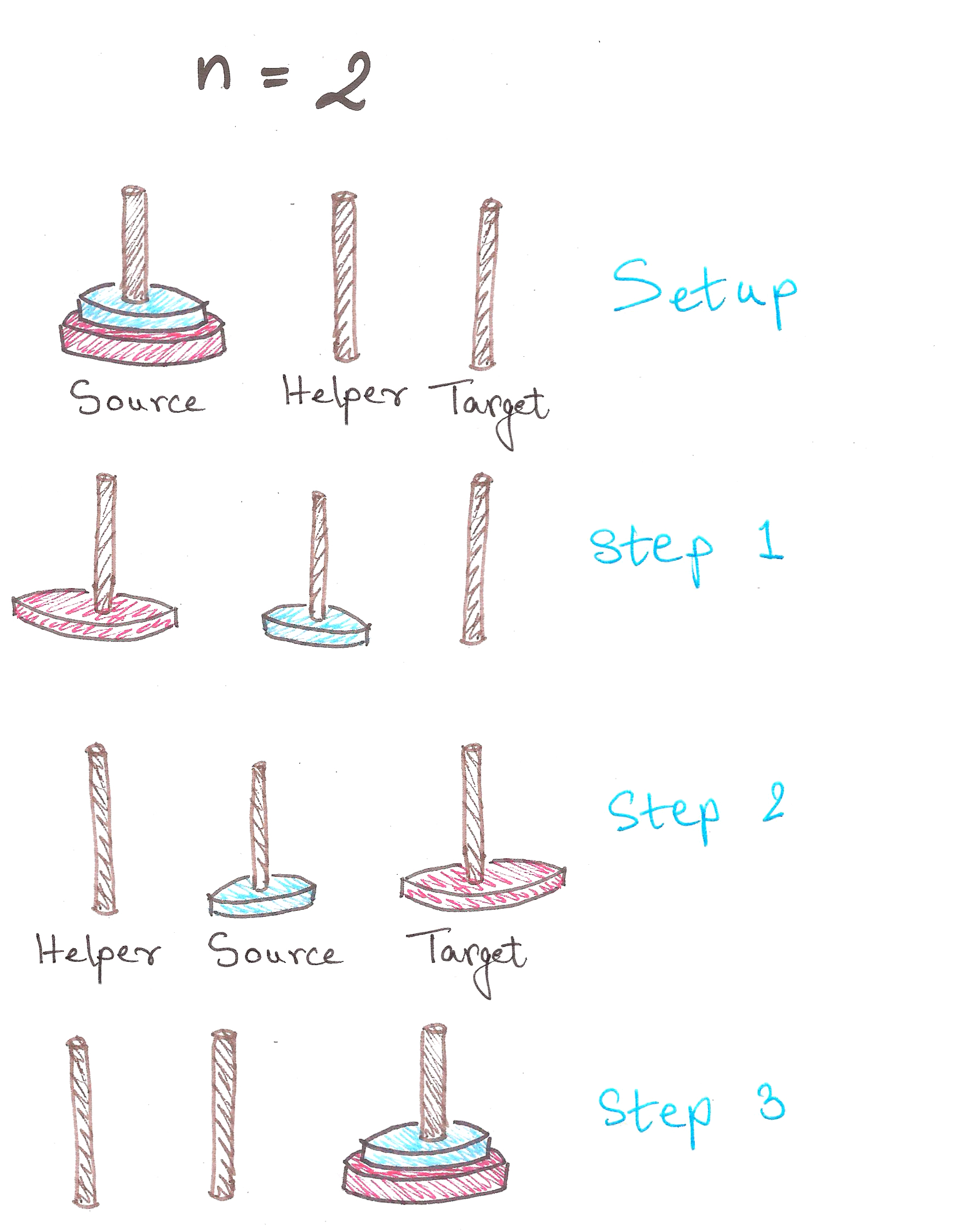3 steps. We’re moving along now.
In Step 1, notice how after we shift the 1st disc to Helper, we have a tower of n – 1 = 1 disc, on Helper.

For n = 3:And we’re done in 7 steps!
Observe that in Step 3, we have a tower of n – 1 = 2 discs, on Helper now. Notice anything similar to the previous case?

In case you haven’t noticed…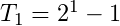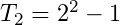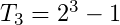In fact, we can prove by mathematical induction, that the least number of moves required to solve a tower of n discs is:Back? Good.

We now need to solve the problem for n = 7. We can of course, figure it out by hand as above, but what’re we learning recursion for then?

A quick recap of the divide-and-conquer method:

Problem
|
Sub-problem (which is a Problem with a smaller input)
|
Base Case (a Problem with a constant output)

So here, in the Tower of Hanoi problem (yeah, that’s the official name), we have:

The general rule for moving towers:Problem: Moving tower of n discs from Source to Target
|
Sub-Problem: Moving tower of n – 1 discs from Source to Helper, then moving last disc to Target
|
Base Case: Moving tower of n = 1

Great. Let’s finally translate this into code.

```def hanoi(n, source, helper, target):
if n > 0:
hanoi(n - 1, source, target, helper)    #moving tower of size n - 1 to helper:
#moving disk from source peg to target peg
if source:    #checks whether source is empty or not
target.append(source.pop())    #appends the last disc from source, to target
hanoi(n - 1, helper, source, target)    #moving tower of size n-1 from helper to target

source = [4,3,2,1]
target = []
helper = []
hanoi(len(source),source,helper,target)

print source, helper, target
```Feel free to go through this entire section once more to digest it, because it’s pretty mentally intensive if you aren’t yet used to thinking this way.

Proof by induction:

The base cases for this problem are pretty simple…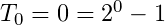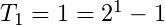Let’s assume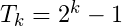to be true for now.

To prove: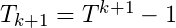is true.

So we have,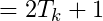Self-similarity is symmetry across scale. It implies recursion, pattern inside of pattern. Monstrous shapes like the Koch Curve display self-similarity because they look exactly the same, even under high magnification. Self-similarity is an easily recognizable quality. It’s images are everywhere in the culture: in the infinitely deep reflection of a person standing between two mirrors, or in the cartoon notion of a fish eating a smaller fish eating a smaller fish eating a smaller fish.

– Chaos – The Amazing Science of the Unpredictable, James Gleick

In 1976, a biologist named Robert May published a seminal paper on the population of animals. This paper would soon create a storm, and not just in biology.

Let’s take a look at what it was all about.

May proposed that variation in population of animals could be modeled by a simple equation, formally called the logistic equation: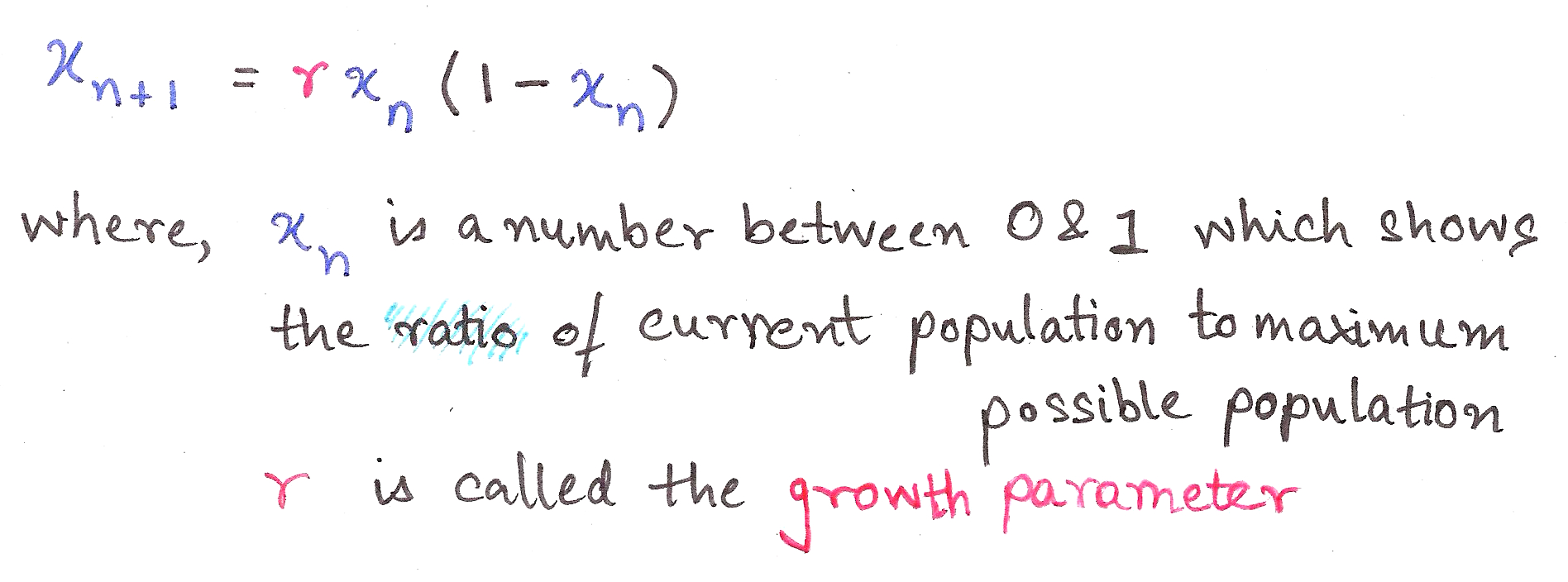This is a recurrence relation, an equation which recursively spits out values as a function of its previous values, ie. takes the output and shoves it back into the input.

It’s such a cute equation, isn’t it? Who’d guess that its interpretation could transform into *this*…This is called a bifurcation diagram, and is also a kind of fractal. It shows the values the population of the species evaluates to finally (as time tends to infinity), depending on the value of r, the growth parameter.

Let’s dissect this.

r between 0 and 1 – Population dies out
r between 1 and 3 – Population converges to a single value
r between 3 and 3.44 – Population oscillates between two values
r between 3.44 and 3.54 – Oscillates between four values
r between 3.54 and 3.56 – Oscillates between 8 values

All of the above are pretty much independent of initial conditions, ie. the initial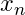we take.

Chaos.

Slight differences in initial conditions result in dramatic changes in population, with no particular order. (In science-y language, ‘Extreme sensitivity to initial conditions’)

This particular paper was path-breaking because it showed how Chaos Theory and fractals aren’t obscure theoretical constructs; they’re things which explain real-life phenomena and its glorious imperfections.

This is a bit beyond the scope of this article, but it goes to show how wide-ranging a role does Recursion play, not just in computer science, but in nature itself.I hope you now have enough a grasp on thinking recursively to understand algorithms like Quick Sort and Merge Sort, and attempt a few basic ad-hoc problems from Codechef et al.

If you are just as fascinated as I am about recursion, then you might enjoy reading about the following:
Fractals
Chaos Theory
Infinite Series and Loops
Feedback loops in Op-amps (they’re an essential part of electronics)

I plan on writing more about the above topics, so do subscribe to Algosaurus for updates.

If you liked this guide, want to insult me, or if you want to talk about dinosaurs or whatever, shoot me a mail at rawrr@algosaur.us

Acknowledgements:
http://www.python-course.eu/towers_of_hanoi.php
https://en.wikipedia.org/wiki/Logistic_map

## 2 thoughts on ““To iterate is human, to recurse, divine.” – A guide to Recursion”

1.Jared Dobson says:

Nice job!!! This is great!2.Rupal Jain says:

As expected, amazing!